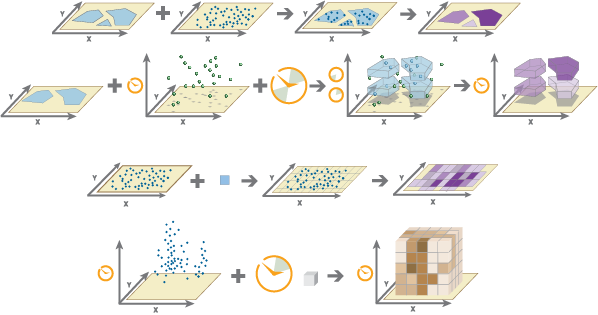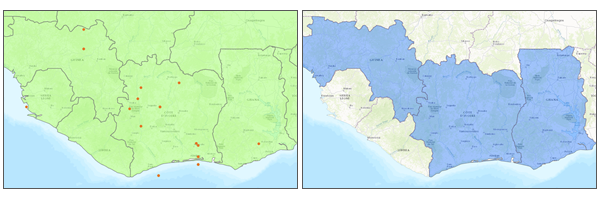# Aggregate Points (GeoAnalytics Desktop)

## Summary

Aggregates points into polygon features or bins. A polygon is returned with a count of points as well as optional statistics at all locations where points exist.

## Illustration## Usage

• The two ways to specify the areas into which the points will be aggregated are as follows:

• Use a polygon layer.
• Use a square or hexagonal bin of a specified size that is generated when the analysis is run.

You can provide the area layer to use for analysis, or you can generate bins of a specified size and shape (hexagon or square) into which to aggregate. The bin size specifies how large the bins are. If you are aggregating into hexagons, the size is the height of each hexagon, and the width of the resulting hexagon will be 2 times the height divided by the square root of 3. If you are aggregating into squares, the bin size is the height of the square, which is equal to the width.

• Analysis with binning requires that your input is projected or that the output coordinate system is set to a projected coordinate system. If your data is not in a projected coordinate system and you do not set one, a projection will be used based on the extent of the data you are analyzing.

• The output feature layer is always a polygon layer. Only polygons that contain points will be returned, and resulting polygons will be completely removed from the result layer.The input point and polygon features (left) and the resulting area features (right) from Aggregate Points are shown.

• If time is enabled on the input, you can apply time stepping to your analysis. Each time step is analyzed independent of features outside the time step. To use time stepping, your input data must be time enabled and represent an instant in time. When time stepping is applied, output features will be time intervals represented by the START_DATETIME and END_DATETIME fields.

• The most basic aggregations will calculate a count of the number of points in each polygon. Statistics (count, sum, minimum, maximum, range, mean, standard deviation, and variance) can also be calculated on numerical fields, and statistics (count, any) can be calculated on string fields. The statistics will be calculated on each area separately. If you specify a statistic that is not valid (mean of a string field), it will be skipped.

##### Note:

When count is applied to a field, it returns a count of the nonnull values present in the field. When any is applied to a string field, it returns a single string present in the field.

• If time is enabled on the input, you can apply time stepping to your analysis. Each time step is analyzed independent of features outside the time step. To use time stepping, your input data must be time enabled and represent an instant in time. When time stepping is applied, output features will be time intervals represented by the START_DATETIME and END_DATETIME fields.

• If you specify a time-step interval, time-step repeat, or reference time, and time is not enabled on the data, the tool will result in an error.

• The Time Step Reference parameter can be a date and time value or solely a date value; it cannot be solely a time value.

• The following fields are included in the output features:

Field nameDescription

count

The count of features within each polygon.

statistic_fieldname

Specified statistics will each create an attribute field, named in the following format: statistic_fieldname. For example, the maximum and standard deviation of the id field are MAX_id and SD_id, respectively.

start_date

When time stepping is specified, output polygons will have a time interval. This field represents the start time.

end_date

When time stepping is specified, output polygons will have a time interval. This field represents the end time.

• You can improve the performance of the Aggregate Points tool by using one or more of the following tips:

• Set the extent environment so you only analyze data of interest.
• Larger bins will perform better than smaller bins. If you are unsure about which size to use, start with a larger bin to prototype.
• Similar to bins, larger time steps will perform better than smaller time steps.
• Use data that is local to where the analysis is being run.

• When running GeoAnalytics Desktop tools, the analysis is completed on your desktop machine. For optimal performance, data should be available on your desktop. If you are using a hosted feature layer, it is recommended that you use ArcGIS GeoAnalytics Server. If your data isn't local, it will take longer to run a tool. To use your ArcGIS GeoAnalytics Server to perform analysis, see GeoAnalytics Tools.

• Similar analysis can also be completed using the Aggregate Points tool in the Standard Feature Analysis toolbox.

## Syntax

`AggregatePoints(point_layer, out_feature_class, polygon_or_bin, {polygon_layer}, {bin_type}, {bin_size}, {time_step_interval}, {time_step_repeat}, {time_step_reference}, {summary_fields})`
 Parameter Explanation Data Type point_layer The point features to be aggregated into polygons or bins. Feature Layer out_feature_class A new feature class with the aggregated polygon results. Feature Class polygon_or_bin Specifies how the point_layer will be aggregated.POLYGON —The point layer will be aggregated into a polygon dataset.BIN —The point layer will be aggregated into square or hexagonal bins that are generated when the tool is run. String polygon_layer(Optional) The polygon features into which the input points will be aggregated. Feature Layer bin_type(Optional) Specifies the bin shape that will be generated to hold the aggregated points.SQUARE —Square bins will be generated. in which bin_size represents the height of a square. This is the default.HEXAGON —Hexagonal bins will be generated, in which bin_size represents the height between two parallel sides. String bin_size(Optional) The distance interval that represents the bin size and units into which the point_layer will be aggregated. The distance interval must be a linear unit. Linear Unit time_step_interval(Optional) A value that specifies the duration of the time step. This parameter is only available if the input points are time enabled and represent an instant in time. Time stepping can only be applied if time is enabled on the input. Time Unit time_step_repeat(Optional) A value that specifies how often the time-step interval occurs. This parameter is only available if the input points are time enabled and represent an instant in time. Time Unit time_step_reference(Optional) A date that specifies the reference time with which to align the time steps. The default is January 1, 1970, at 12:00 a.m. This parameter is only available if the input points are time enabled and represent an instant in time. Date summary_fields[summary_fields,...](Optional) The statistics that will be calculated on specified fields. COUNT—The number of nonnull values. It can be used on numeric fields or strings. The count of [null, 0, 2] is 2.SUM—The sum of numeric values in a field. The sum of [null, null, 3] is 3.MEAN—The mean of numeric values. The mean of [0,2, null] is 1.MIN—The minimum value of a numeric field. The minimum of [0, 2, null] is 0.MAX—The maximum value of a numeric field. The maximum value of [0, 2, null] is 2.STDDEV—The standard deviation of a numeric field. The standard deviation of  is null. The standard deviation of [null, 1,1,1] is null.VAR—The variance of a numeric field in a track. The variance of  is null. The variance of [null, 1,1,1] is null.RANGE—The range of a numeric field. This is calculated as the minimum value subtracted from the maximum value. The range of [0, null, 1] is 1. The range of [null, 4] is 0.ANY—A sample string from a field of type string. Value Table

## Code sample

AggregatePoints (Python window)

The following Python window script demonstrates how to use the AggregatePoints tool.

``````#-------------------------------------------------------------------------------
# Name: AggregatePoints.py
# Description: Aggregate 311 events into 1 KM Bins.

# Import system modules
import arcpy

arcpy.env.workspace = "C:/data/CityData.gdb"

# Set local variables
inFeatures = "SF311"
summaryFields = ["Year", "Beat"]
summaryStatistics = [["Arrest", "COUNT"], ["District", "COUNT"]]
out = "AggregateWildfires"

# Execute Aggregate Points
arcpy.gapro.AggregatePoints(inFeatures, out, "BIN", None, "HEXAGON",
"1 Kilometers")``````

• Basic: No
• Standard: No